# PyDiffGame is a Python implementation of a Nash Equilibrium solution to Differential Games, based on a reduction of Game Hamilton-Bellman-Jacobi (GHJB) equations to Game Algebraic and Differential Riccati equations, associated with Multi-Objective Dynamical Control Systemskrichelj, updated 🕥 2023-03-17 16:47:04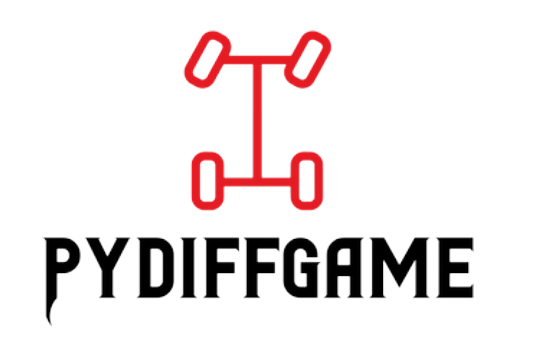# What is this?

PyDiffGame is a Python implementation of a Nash Equilibrium solution to Differential Games, based on a reduction of Game Hamilton-Bellman-Jacobi (GHJB) equations to Game Algebraic and Differential Riccati equations, associated with Multi-Objective Dynamical Control Systems\ The method relies on the formulation given in:

• The thesis work "Differential Games for Compositional Handling of Competing Control Tasks" (Research Gate)

• The conference article "Composition of Dynamic Control Objectives Based on Differential Games" (IEEE | Research Gate)

If you use this work, please cite our paper: @conference{med_paper, author={Kricheli, Joshua Shay and Sadon, Aviran and Arogeti, Shai and Regev, Shimon and Weiss, Gera}, booktitle={29th Mediterranean Conference on Control and Automation (MED)}, title={{Composition of Dynamic Control Objectives Based on Differential Games}}, year={2021}, pages={298-304}, doi={10.1109/MED51440.2021.9480269}}

# Installation

To install this package run this from the command prompt: pip install PyDiffGame

The package was tested for Python >= 3.10, along with the listed packages versions in requirments.txt

# Input Parameters

The package defines an abstract class PyDiffGame.py. An object of this class represents an instance of differential game. The input parameters to instantiate a PyDiffGame object are:

• A : np.array of shape $(n,n)$

System dynamics matrix

• B : np.array of shape( $n,\sum_{i=1}^N m_i$ ), optional

Input matrix for all virtual control objectives

• Bs : Sequence of np.array objects of len $(N)$, each array $B_i$ of shape $(n,m_i)$, optional

Input matrices for each virtual control objective

• Qs : Sequence of np.array objects of len $(N)$, each array $Q_i$ of shape $(n,n)$, optional

State weight matrices for each virtual control objective

• Rs : Sequence of np.array objects of len $(N)$, each array $R_i$ of shape $(m_i,m_i)$, optional

Input weight matrices for each virtual control objective

• Ms : Sequence of np.array objects of len $(N)$, each array $M_i$ of shape $(m_i,m)$, optional

Decomposition matrices for each virtual control objective

• objectives : Sequence of Objective objects of len $(N)$, each $O_i$ specifying $Q_i, R_i$ and $M_i$, optional

Desired objectives for the game

• x_0 : np.array of len $(n)$, optional

Initial state vector

• x_T : np.array of len $(n)$, optional

Final state vector, in case of signal tracking

• T_f : positive float, optional

System dynamics horizon. Should be given in the case of finite horizon

• P_f : list of np.array objects of len $(N)$, each array $P_{f_i}$ of shape $(n,n)$, optional, default = uncoupled solution of scipy's solve_are

Final condition for the Riccati equation array. Should be given in the case of finite horizon

• state_variables_names : Sequence of str objects of len $(N)$, optional

The state variables' names to display when plotting

• show_legend : boolean, optional

Indicates whether to display a legend in the plots

• state_variables_names : Sequence of str objects of len $(n)$, optional

The state variables' names to display

• epsilon_x : float in the interval $(0,1)$, optional

Numerical convergence threshold for the state vector of the system

• epsilon_P : float in the interval $(0,1)$, optional

Numerical convergence threshold for the matrices P_i

• L : positive int, optional

Number of data points

• eta : positive int, optional

The number of last matrix norms to consider for convergence

• debug : boolean, optional

Indicates whether to display debug information

# Tutorial

To demonstrate the use of the package, we provide a few running examples. Consider the following system of masses and springs:The performance of the system under the use of the suggested method is compared with that of a Linear Quadratic Regulator (LQR). For that purpose, class named PyDiffGameLQRComparison is defined. A comparison of a system should subclass this class. As an example, for the masses and springs system, consider the following instantiation of an MassesWithSpringsComparison object:

python import numpy as np from PyDiffGame.examples.MassesWithSpringsComparison import MassesWithSpringsComparison

N = 2 k = 10 m = 50 r = 1 epsilon_x = 10e-8 epsilon_P = 10e-8 q = [[500, 2000], [500, 250]]

x_0 = np.array([10 * i for i in range(1, N + 1)] +  * N) x_T = x_0 * 10 if N == 2 else np.array([(10 * i) ** 3 for i in range(1, N + 1)] +  * N) T_f = 25

masses_with_springs = MassesWithSpringsComparison(N=N, m=m, k=k, q=q, r=r, x_0=x_0, x_T=x_T, T_f=T_f, epsilon_x=epsilon_x, epsilon_P=epsilon_P) 

Consider the constructor of the class MassesWithSpringsComparison:

python import numpy as np from typing import Sequence, Optional

from PyDiffGame.PyDiffGame import PyDiffGame from PyDiffGame.PyDiffGameLQRComparison import PyDiffGameLQRComparison from PyDiffGame.Objective import GameObjective, LQRObjective

class MassesWithSpringsComparison(PyDiffGameLQRComparison): def init(self, N: int, m: float, k: float, q: float | Sequence[float] | Sequence[Sequence[float]], r: float, Ms: Optional[Sequence[np.array]] = None, x_0: Optional[np.array] = None, x_T: Optional[np.array] = None, T_f: Optional[float] = None, epsilon_x: Optional[float] = PyDiffGame.epsilon_x_default, epsilon_P: Optional[float] = PyDiffGame.epsilon_P_default, L: Optional[int] = PyDiffGame.L_default, eta: Optional[int] = PyDiffGame.eta_default): I_N = np.eye(N) Z_N = np.zeros((N, N))

    M_masses = m * I_N
K = k * (2 * I_N - np.array([[int(abs(i - j) == 1) for j in range(N)] for i in range(N)]))
M_masses_inv = np.linalg.inv(M_masses)

M_inv_K = M_masses_inv @ K

if Ms is None:
eigenvectors = np.linalg.eig(M_inv_K)
Ms = [eigenvector.reshape(1, N) for eigenvector in eigenvectors]

A = np.block([[Z_N, I_N],
[-M_inv_K, Z_N]])
B = np.block([[Z_N],
[M_masses_inv]])

Qs = [np.diag([0.0] * i + [q] + [0.0] * (N - 1) + [q] + [0.0] * (N - i - 1))
if isinstance(q, (int, float)) else
np.diag([0.0] * i + [q[i]] + [0.0] * (N - 1) + [q[i]] + [0.0] * (N - i - 1)) for i in range(N)]

M = np.concatenate(Ms,
axis=0)

assert np.all(np.abs(np.linalg.inv(M) - M.T) < 10e-12)

Q_mat = np.kron(a=np.eye(2),
b=M)

Qs = [Q_mat.T @ Q @ Q_mat for Q in Qs]

Rs = [np.array([r])] * N
R_lqr = 1 / 4 * r * I_N
Q_lqr = q * np.eye(2 * N) if isinstance(q, (int, float)) else np.diag(2 * q)

state_variables_names = ['x_{' + str(i) + '}' for i in range(1, N + 1)] + \
['\\dot{x}_{' + str(i) + '}' for i in range(1, N + 1)]
args = {'A': A,
'B': B,
'x_0': x_0,
'x_T': x_T,
'T_f': T_f,
'state_variables_names': state_variables_names,
'epsilon_x': epsilon_x,
'epsilon_P': epsilon_P,
'L': L,
'eta': eta}

lqr_objective = [LQRObjective(Q=Q_lqr,
R_ii=R_lqr)]
game_objectives = [GameObjective(Q=Q,
R_ii=R,
M_i=M_i) for Q, R, M_i in zip(Qs, Rs, Ms)]
games_objectives = [lqr_objective,
game_objectives]

super().__init__(args=args,
M=M,
games_objectives=games_objectives,
continuous=True)




Finally, consider calling the masses_with_springs object as follows:

python output_variables_names = ['$\frac{x_1 + x_2}{\sqrt{2}}$', '$\frac{x_2 - x_1}{\sqrt{2}}$', '$\frac{\dot{x}_1 + \dot{x}_2}{\sqrt{2}}$', '$\frac{\dot{x}_2 - \dot{x}_1}{\sqrt{2}}$']

masses_with_springs(plot_state_spaces=True, plot_Mx=True, output_variables_names=output_variables_names, save_figure=True) 

Refer This will result in the following plot that compares the two systems performance for a differential game vs an LQR: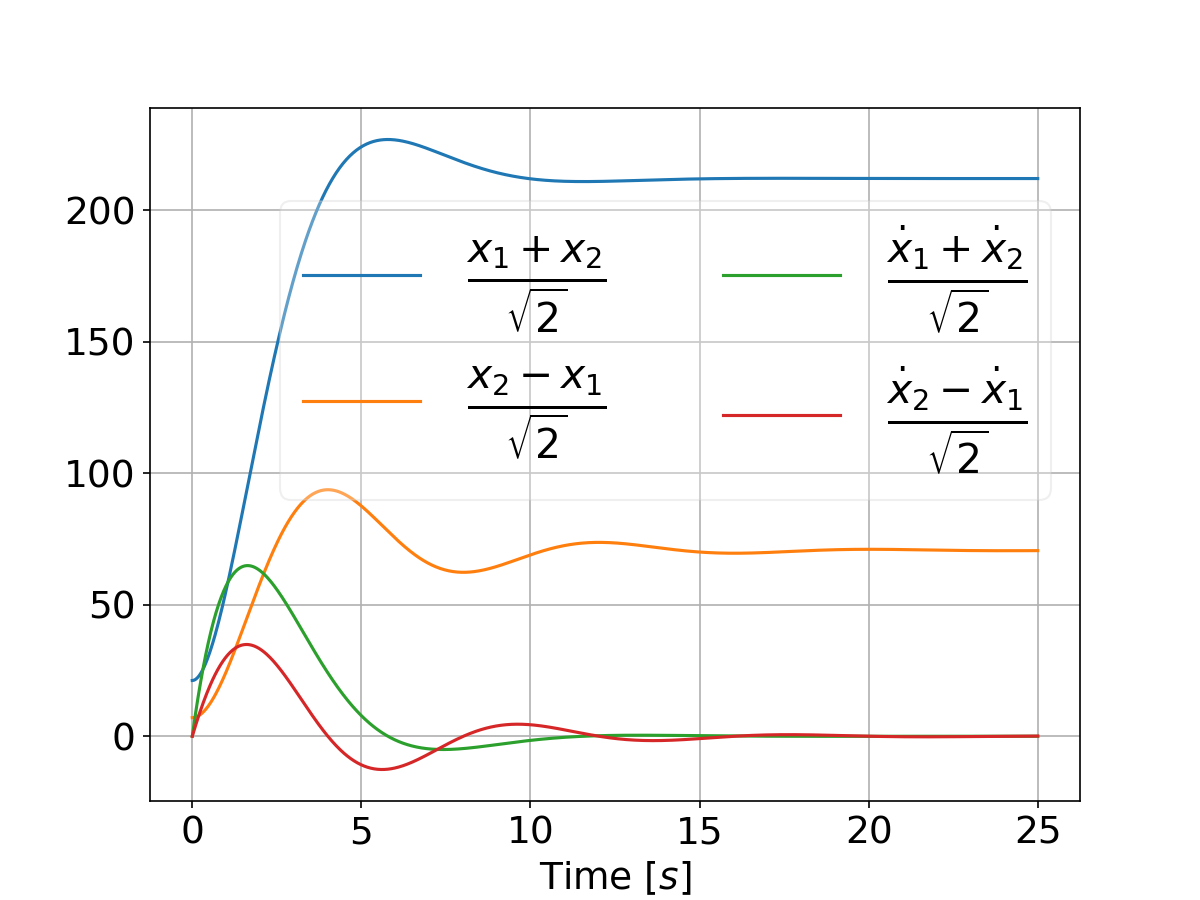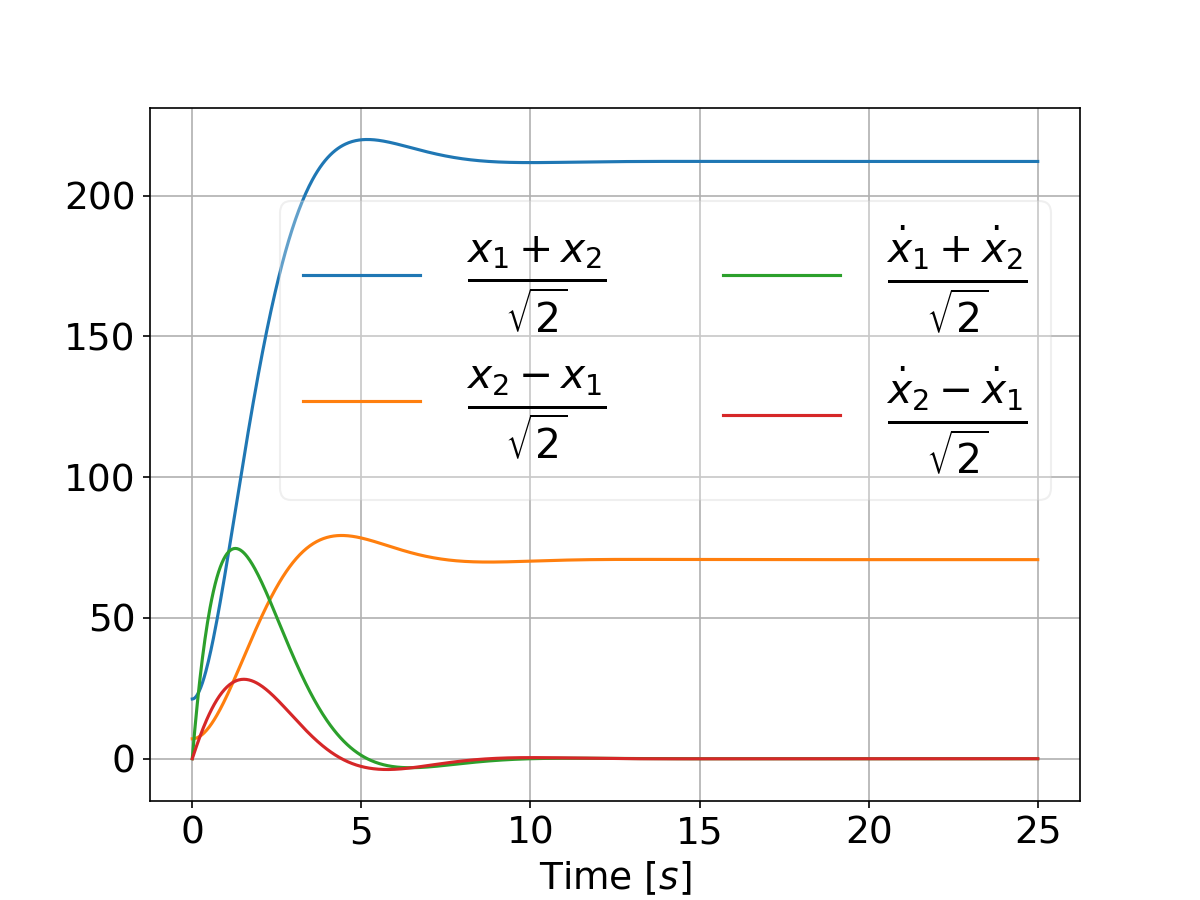And when tweaking the weights by setting

python qs = [[500, 5000]]

we have: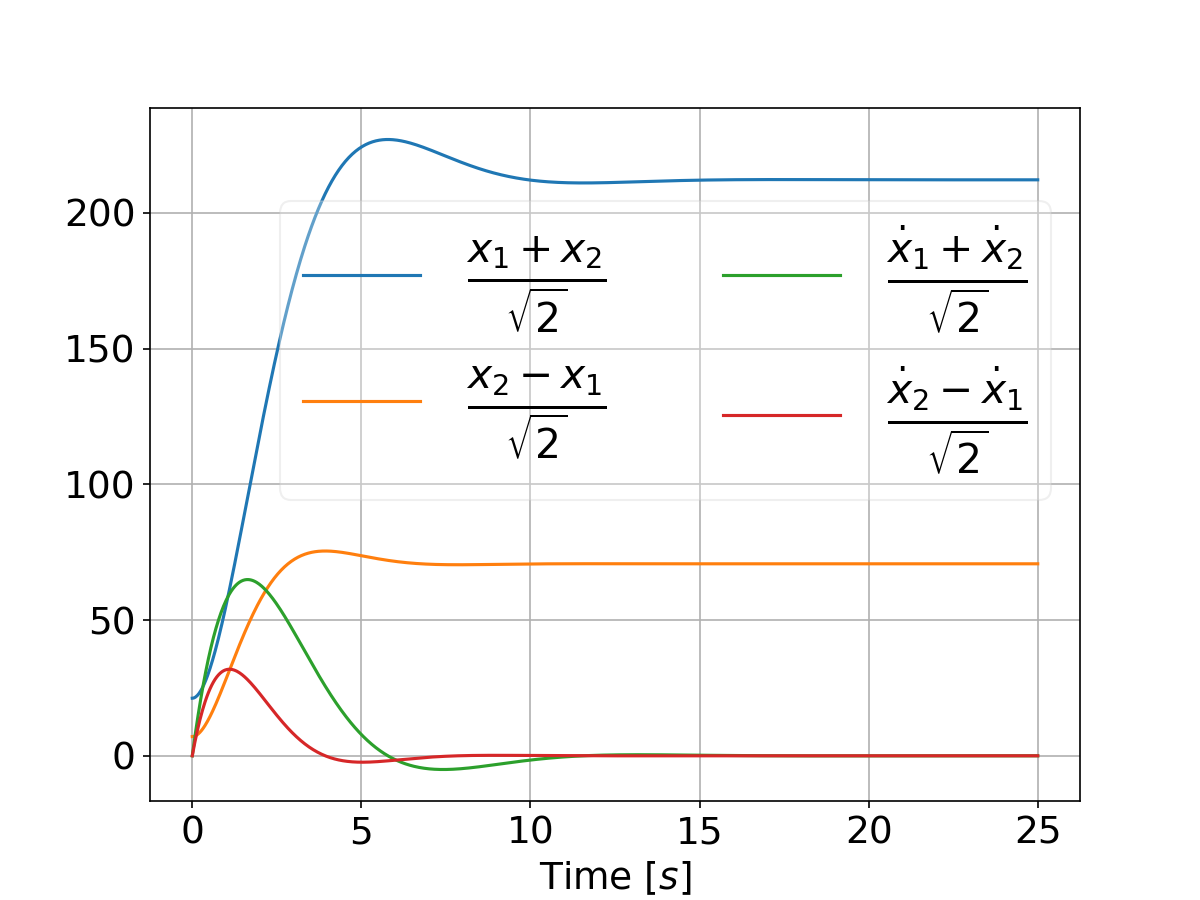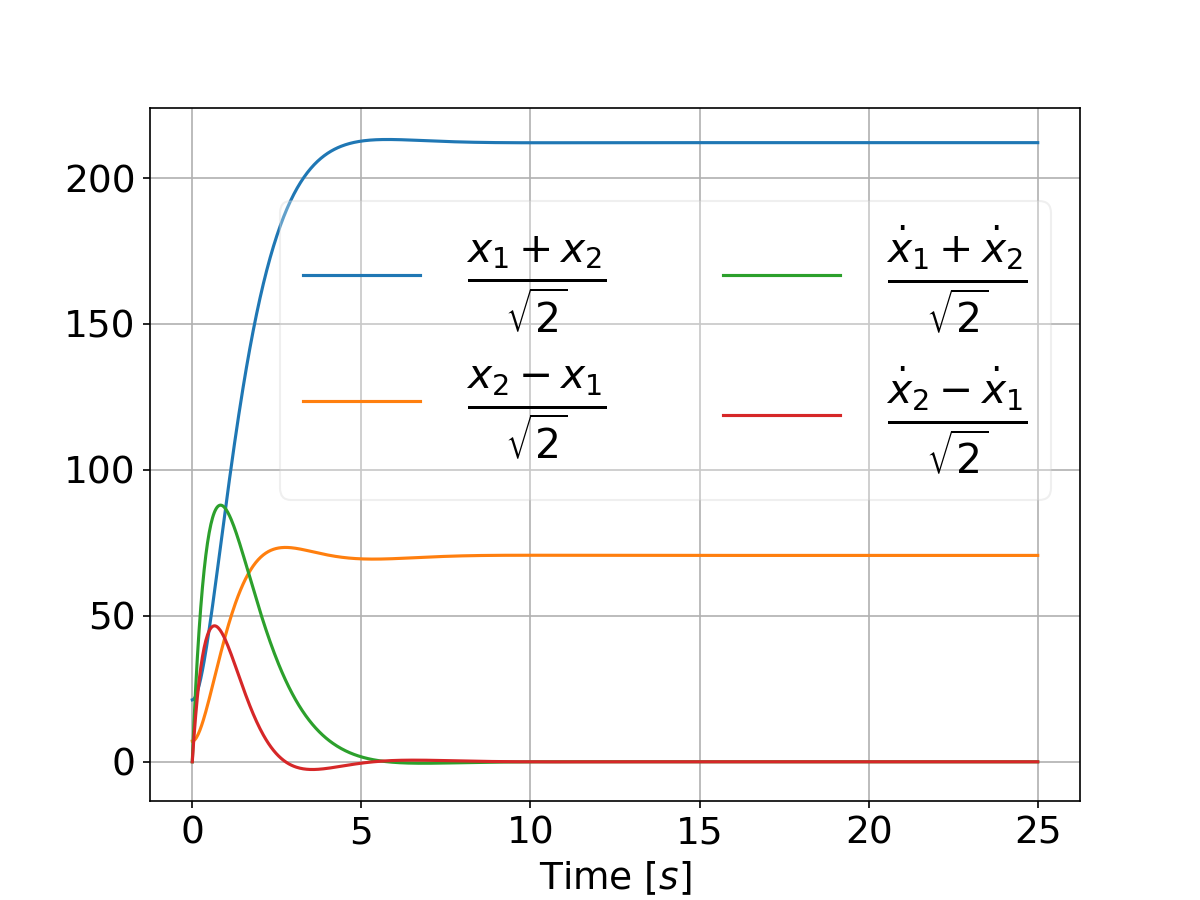# Acknowledgments

This research was supported in part by the Helmsley Charitable Trust through the Agricultural, Biological and Cognitive Robotics Initiative and by the Marcus Endowment Fund both at Ben-Gurion University of the Negev, Israel. This research was also supported by The Israeli Smart Transportation Research Center (ISTRC) by The Technion and Bar-Ilan Universities, Israel.

## Releases

### v0.1.1 2023-02-28 10:48:23

Uploaded as a package to PyPI

### v0.1.0 2023-02-17 11:07:48

Initial release

#####Joshua Shay Kricheli

AI/ML Researcher, Vice President Data Science, M.Sc. in Computer Science, B.Sc. in Mechanical Engineering

control-theory riccati-equation differential-game quadrotor multi-objective-optimization matrix-equations differential-equations game-theory bellman-equation hamiltonian pontryagin-maximum-principle hamilton-jacobi-bellman dynamical-systems nash-equilibrium artificial-intelligence-algorithms numerical-methods inverted-pendulum optimization-algorithms object-oriented-programming computer-science